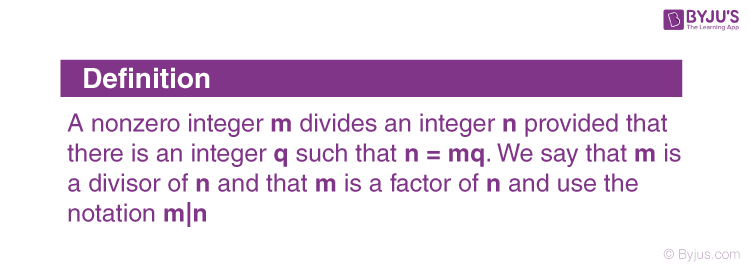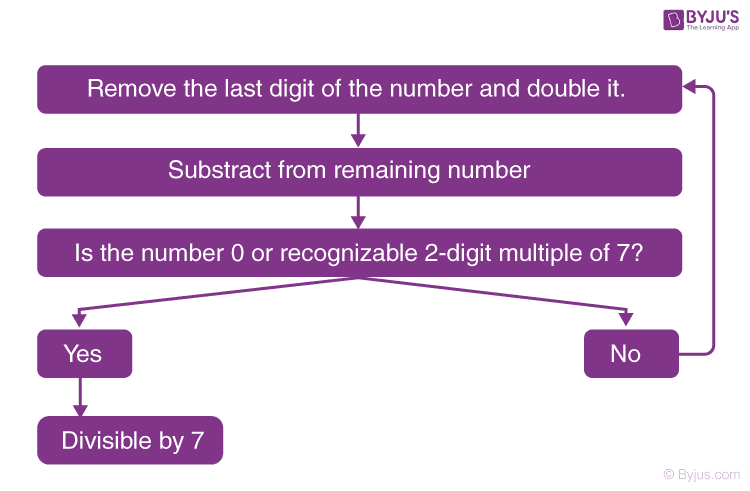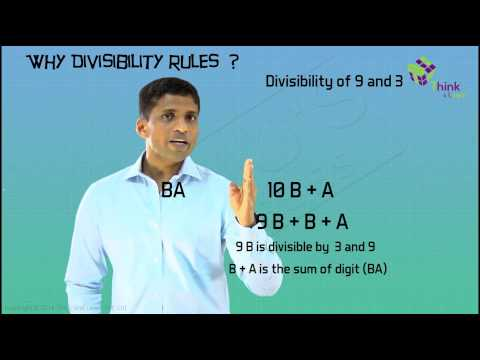Checkout JEE MAINS 2022 Question Paper Analysis : Checkout JEE MAINS 2022 Question Paper Analysis :

# Divisibility Rules

Divisibility rules or Divisibility tests have been mentioned to make the division procedure easier and quicker. If students learn the division rules in Maths or the divisibility tests for 1 to 20, they can solve the problems in a better way. For example, divisibility rules for 13 help us to know which numbers are completely divided by 13. Some numbers like 2, 3, 4, 5 have rules which can be understood easily. But rules for 7, 11, 13, are a little complex and need to be understood in-depth.

Mathematics is not easy for some of us. At times, the need for tricks and shorthand techniques is felt, to solve Maths problems faster and easily without lengthy calculation. It will also help students to score better marks in exams. These rules are a great example of such shorthand techniques. In this article, let us discuss the division rules in Maths with many examples.

## Divisibility Test (Division Rules in Maths)

As the name suggests, divisibility tests or division rules in Maths help one to check whether a number is divisible by another number without the actual method of division. If a number is completely divisible by another number then the quotient will be a whole number and the remainder will be zero.Since every number is not completely divisible by every other number such numbers leave remainder other than zero. These rules are certain ones, which help us to determine the actual divisor of a number just by considering the digits of the number.

The division rules from 1 to 13 in Maths are explained here in detail with many solved examples. Go through the below article to learn the shortcut methods to divide the numbers easily.

### Divisibility Rule of 1

Every number is divisible by 1. Divisibility rule for 1 doesn’t have any condition. Any number divided by 1 will give the number itself, irrespective of how large the number is. For example, 3 is divisible by 1 and 3000 is also divisible by 1 completely.

### Divisibility Rule of 2

If a number is even or a number whose last digit is an even number i.e.  2,4,6,8 including 0, it is always completely divisible by 2.

Example: 508 is an even number and is divisible by 2 but 509 is not an even number, hence it is not divisible by 2. Procedure to check whether 508 is divisible by 2 or not is as follows:

• Consider the number 508
• Just take the last digit 8 and divide it by 2
• If the last digit 8 is divisible by 2 then the number 508 is also divisible by 2.

### Divisibility Rules for 3

Divisibility rule for 3 states that a number is completely divisible by 3 if the sum of its digits is divisible by 3.

Consider a number, 308. To check whether 308 is divisible by 3 or not, take sum of the digits (i.e. 3+0+8= 11). Now check whether the sum is divisible by 3 or not. If the sum is a multiple of 3, then the original number is also divisible by 3. Here, since 11 is not divisible by 3, 308 is also not divisible by 3.

Similarly, 516 is divisible by 3 completely as the sum of its digits i.e. 5+1+6=12, is a multiple of 3.

### Divisibility Rule of 4

If the last two digits of a number are divisible by 4, then that number is a multiple of 4 and is divisible by 4 completely.

Example: Take the number 2308. Consider the last two digits i.e.  08. As 08 is divisible by 4, the original number 2308 is also divisible by 4.

### Divisibility Rule of 5

Numbers, which last with digits, 0 or 5 are always divisible by 5.
Example: 10, 10000, 10000005, 595, 396524850, etc.

### Divisibility Rule of 6

Numbers which are divisible by both 2 and 3 are divisible by 6. That is, if the last digit of the given number is even and the sum of its digits is a multiple of 3, then the given number is also a multiple of 6.

Example: 630, the number is divisible by 2 as the last digit is 0.
The sum of digits is 6+3+0 = 9, which is also divisible by 3.
Hence, 630 is divisible by 6.

### Divisibility Rules for 7

The rule for divisibility by 7 is a bit complicated which can be understood by the steps given below:Example: Is 1073 divisible by 7?

• From the rule stated remove 3 from the number and double it, which becomes 6.
• Remaining number becomes 107, so 107-6 = 101.
• Repeating the process one more time, we have 1 x 2 = 2.
• Remaining number 10 – 2 = 8.
• As 8 is not divisible by 7, hence the number 1073 is not divisible by 7.

### Divisibility Rule of 8

If the last three digits of a number are divisible by 8, then the number is completely divisible by 8.

Example: Take number 24344. Consider the last two digits i.e.  344. As 344 is divisible by 8, the original number 24344 is also divisible by 8.

### Divisibility Rule of 9

The rule for divisibility by 9 is similar to divisibility rule for 3. That is, if the sum of digits of the number is divisible by 9, then the number itself is divisible by 9.

Example: Consider 78532, as the sum of its digits (7+8+5+3+2) is 25, which is not divisible by 9, hence 78532 is not divisible by 9.

### Divisibility Rule of 10

Divisibility rule for 10 states that any number whose last digit is 0, is divisible by 10.

Example: 10, 20, 30, 1000, 5000, 60000, etc.

### Divisibility Rules for 11

If the difference of the sum of alternative digits of a number is divisible by 11, then that number is divisible by 11 completely.

In order to check whether a number like 2143 is divisible by 11, below is the following procedure.

• Group the alternative digits i.e. digits which are in odd places together and digits in even places together. Here 24 and 13 are two groups.
• Take the sum of the digits of each group i.e. 2+4=6 and 1+3= 4
• Now find the difference of the sums; 6-4=2
• If the difference is divisible by 11, then the original number is also divisible by 11. Here 2 is the difference which is not divisible by 11.
• Therefore, 2143 is not divisible by 11.

A few more conditions are there to test the divisibility of a number by 11. They are explained here with the help of examples:

If the number of digits of a number is even, then add the first digit and subtract the last digit from the rest of the number.

Example: 3784

Number of digits = 4

Now, 78 + 3 – 4 = 77 = 7 × 11

Thus, 3784 is divisible by 11.

If the number of digits of a number is odd, then subtract the first and the last digits from the rest of the number.

Example: 82907

Number of digits = 5

Now, 290 – 8 – 7 = 275 × 11

Thus, 82907 is divisible by 11.

Form the groups of two digits from the right end digit to the left end of the number and add the resultant groups. If the sum is a multiple of 11, then the number is divisible by 11.

Example: 3774 := 37 + 74 = 111 := 1 + 11 = 12

3774 is not divisible by 11.

253 := 2 + 53 = 55 = 5 × 11

253 is divisible by 11.

Subtract the last digit of the number from the rest of the number. If the resultant value is a multiple of 11, then the original number will be divisible by 11.

Example: 9647

9647 := 964 – 7 = 957

957 := 95 – 7 = 88 = 8 × 11

Thus, 9647 is divisible by 11.

### Divisibility Rule of 12

If the number is divisible by both 3 and 4, then the number is divisible by 12 exactly.

Example: 5864

Sum of the digits = 5 + 8 + 6 + 4 = 23 (not a multiple of 3)

Last two digits = 64 (divisible by 4)

The given number 5846 is divisible by 4 but not by 3; hence, it is not divisible by 12.

### Divisibility Rules for 13

For any given number, to check if it is divisible by 13, we have to add four times of the last digit of the number to the remaining number and repeat the process until you get a two-digit number.  Now check if that two-digit number is divisible by 13 or not. If it is divisible, then the given number is divisible by 13.

For example: 2795 → 279 + (5 x 4)

→ 279 + (20)

→ 299

→ 29 + (9 x 4)

→ 29 + 36

→65

Number 65 is divisible by 13, 13 x 5 = 65.

## Video Lesson

### Divisibility Models## Solved Examples

Example 1:

Check if 288 is divisible by 2.

Solution:

Given, 288 is a number.

If the last digit of 288 is divisible by 2, then 288 is also divisible by 2.

The last digit of 288 is 8, which is divisible by 2, such that;

8/2 = 4

Hence, 288 satisfy the divisibility rule for 2.

Example 2:

Check is 195 is divisible by 4 or not.

Solution:

As we can see, the last digit of 195 is 5, which is not divisible by 4.

Hence, 195 is not divisible by 4.## Frequently Asked Questions on Divisibility Rules

### What is meant by divisibility rules?

A divisibility test is an easy way to identify whether the given number is divided by a fixed divisor without actually performing the division process. If a number is completely divided by another number, then the quotient should be a whole number and the remainder should be zero.

### What is the divisibility rule for 2 and 5?

Divisibility rule for 2: The last/unit digit of the given number should be an even number or the multiples of 2. (i.e.,) 0, 2, 4, 6, and 8.
Divisibility Rule for 5: The unit digit of the given number should be 0 or 5.

### What is the divisibility rule for 7 and give an example?

The difference between twice the unit digit of the given number and the remaining part of the given number should be a multiple of 7 or it should be zero. For example, 147 is divisible by 7. Here, the unit digit is 7. When it is multiplied by 2, we get 14, and the remaining part is 14. Therefore, the difference between 14 and 14 is 0.

### Write down the divisibility rule for 9.

The sum of the digits of the given number should be divisible by 9. For example, 2979 is divisible by 9. (i.e.,) 2+9+7+9 = 27, which is divisible by 9.

### What is the divisibility rule for 13?

The sum/addition of four times of the unit digit and the remaining part of the given number should be a multiple of 13. For example, 1092 is divisible by 13. Here, the unit digit is 2. When it is multiplied by 4, we get 8, and the remaining part of the number is 109. Hence, the sum of 109 and 8 is 117, which is divisible by 13.

Download BYJU’S- The Learning App and get personalised video content explaining different types of mathematical operations such as multiplication, division, etc.

Quiz on Divisibility Rules

1. Mahes.m

Very useful…. clearly explained..

2. Ishmeal s.kollie

I must commend you guys for such a splendid effort. You have done extremely well for providing such a good learning environment. Bravo!

3. b kanak raju

nice rule

4. Aaryan Kumar

it was very useful for me and my exams. THANK YOU BYJU’S.

5. it works well gives good information

6. Nice explaination tq

7. can you help me out with some question on the divisibilty of 7 an 13
It’s urgent

8. Lakshya Dhawan

Thank you so much

9. yatharth

nice teaching sir!!!!!!!!!!!

10. Loryrubina

very helpful .. thank you very much. God Bless.

11. RISHABH

Give one example for each to explain the test of divisibility by 6 and 11

12. Sathish B

Very helpful. Thank you very much.

13. Geethavallika

Very useful thank you byju’s

14. Tejaswinijaiswal

Thanku byjus for helping me

15. Thank you byjus for helping me